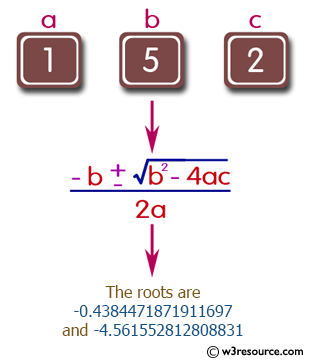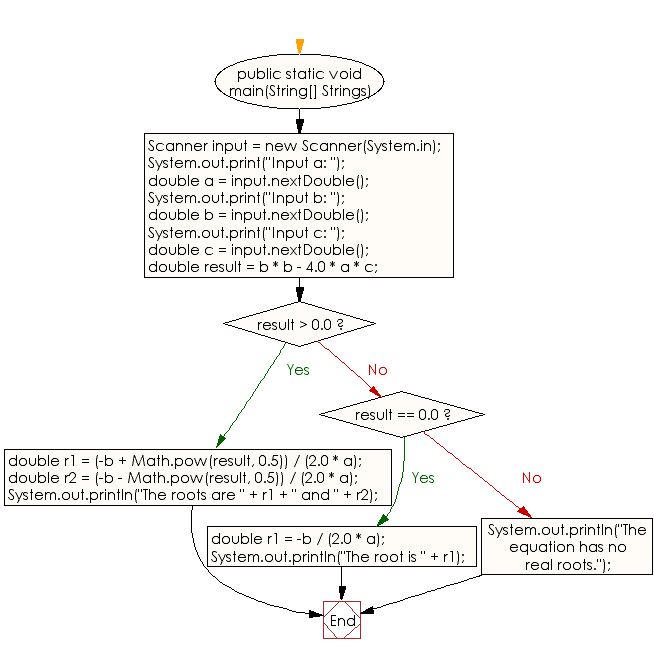﻿ Java exercises: Solve quadratic equations - w3resource# Java Conditional Statement Exercises: Solve quadratic equations

## Java Conditional Statement: Exercise-2 with Solution

Write a Java program to solve quadratic equations (use if, else if and else).

Test Data
Input a: 1
Input b: 5
Input c: 1

Pictorial Presentation:Sample Solution:

Java Code:

``````import java.util.Scanner;
public class Exercise2 {

public static void main(String[] Strings) {

Scanner input = new Scanner(System.in);

System.out.print("Input a: ");
double a = input.nextDouble();
System.out.print("Input b: ");
double b = input.nextDouble();
System.out.print("Input c: ");
double c = input.nextDouble();

double result = b * b - 4.0 * a * c;

if (result > 0.0) {
double r1 = (-b + Math.pow(result, 0.5)) / (2.0 * a);
double r2 = (-b - Math.pow(result, 0.5)) / (2.0 * a);
System.out.println("The roots are " + r1 + " and " + r2);
} else if (result == 0.0) {
double r1 = -b / (2.0 * a);
System.out.println("The root is " + r1);
} else {
System.out.println("The equation has no real roots.");
}

}
}
```
```

Sample Output:

```Input a: 1
Input b: 5
Input c: 2
The roots are -0.4384471871911697 and -4.561552812808831
```

Flowchart:Java Code Editor: